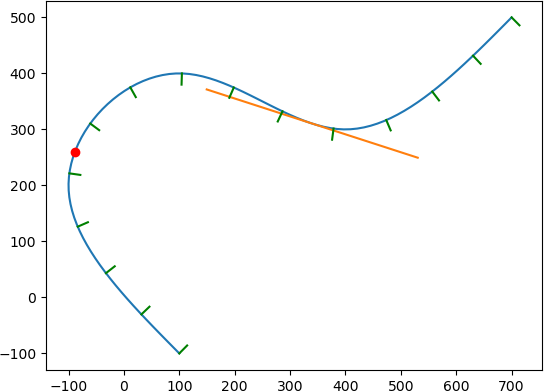TinySpline  0.7.0 Spline Library for a Multitude of Programming Languages
TinySplineTinySpline is a small, yet powerful library for interpolating, transforming, and querying arbitrary NURBS, B-Splines, and Bézier curves. The core of the library is written in ANSI C (C89) with a C++ wrapper for an object-oriented programming model. Based on the C++ wrapper, auto-generated bindings for C#, D, Go, Java, Javascript, Lua, Octave, PHP, Python, R, and Ruby are provided.

## Features

• Object-oriented programming model
• B-Splines of any degree and dimensionality
• Spline interpolation
• Cubic natural
• Centripetal Catmull–Rom
• Evaluation
• Knots
• Sampling (multiple knots at once)
• Equidistant points
• Components (find y for given x)
• Reparametrization by arc length
• Mapping length <--> knot
• Knot insertion (refinement)
• Sub-spline extraction
• Bézier curve decomposition
• (also known as subdivision)
• Derivative
• Degree elevation
• Computation of rotation minimizing frames
• Morphing
• Serialization (JSON)
• Vector math

## Installation

### Pre-built Binaries

Conan (C/C++):
https://conan.io/center/tinyspline

NuGet (C#):

<PackageReference Include="tinyspline" Version="0.6.0.1" />

Go:

go get github.com/tinyspline/go@v0.6.0

Luarocks (Lua):

luarocks install --server=https://tinyspline.github.io/lua tinyspline

Maven (Java):

<dependency>
<groupId>org.tinyspline</groupId>
<artifactId>tinyspline</artifactId>
<version>0.6.0-1</version>
</dependency>

PyPI (Python):

python -m pip install tinyspline

RubyGems (Ruby):

gem install tinyspline

See BUILD.md.

## Getting Started

A variety of examples (tests) can be found in the [test](test) subdirectory.

The following listing shows a Python example:

from tinyspline import *
import matplotlib.pyplot as plt
spline = BSpline.interpolate_cubic_natural(
[
100, -100, # P1
-100, 200, # P2
100, 400, # P3
400, 300, # P4
700, 500 # P5
], 2) # <- dimensionality of the points
# Draw spline as polyline.
points = spline.sample(100)
x = points[0::2]
y = points[1::2]
plt.plot(x, y)
# Draw point at knot 0.3.
vec2 = spline.eval(0.3).result_vec2()
plt.plot(vec2.x, vec2.y, 'ro')
# Draw tangent at knot 0.7.
pos = spline(0.7).result_vec2() # operator () -> eval
der = spline.derive()(0.7).result_vec2().normalize() * 200
s = pos - der
t = pos + der
plt.plot([s.x, t.x], [s.y, t.y])
# Draw 15 normals with equidistant distribution.
knots = spline.equidistant_knot_seq(15)
frames = spline.compute_rmf(knots)
for i in range(frames.size()):
pos = frames.at(i).position
nor = pos + frames.at(i).normal * 20
# You can also fetch the tangent and binormal:
# frames.at(i).tangent
# frames.at(i).binormal
plt.plot([pos.x, nor.x], [pos.y, nor.y], 'g')
plt.show()

Result:## Documentation

The latest Doxygen documentation can be found at: https://msteinbeck.github.io/tinyspline/

The documentation of the C interface (https://msteinbeck.github.io/tinyspline/tinyspline_8h.html) is quite extensive and also serves as an entry point for the C++ interface documentation (as well as the documentation for the bindings created from the C++ interface).

## Publications

If you use TinySpline in your research, please cite it as below.

@INPROCEEDINGS{Steinbeck:SANER:21,
author = {Steinbeck, Marcel and Koschke, Rainer},
booktitle = {2021 IEEE International Conference on Software
Analysis, Evolution and Reengineering (SANER)},
title = {TinySpline: A Small, yet Powerful Library for
Interpolating, Transforming, and Querying NURBS,
B-Splines, and Bézier Curves},
year = {2021},
pages = {572-576},
doi = {10.1109/SANER50967.2021.00068}
}

Other publications:

@INPROCEEDINGS{Steinbeck:VISSOFT:22,
author = {Steinbeck, Marcel and Koschke, Rainer},
booktitle = {2022 Working Conference on Software Visualization
(VISSOFT)},
title = {Edge Animation in Software Visualization},
year = {2022},
pages = {63-74},
doi = {10.1109/VISSOFT55257.2022.00015}
}

## Theoretical Backgrounds

 is a very good starting point for B-Splines.

 explains De Boor's Algorithm and gives some pseudo code.

 provides a good overview of NURBS with some mathematical background.

 is useful if you want to use NURBS in TinySpline.